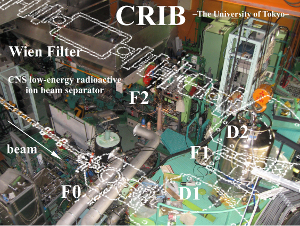=ALL ABOUT CRIB=
CRIB Magnets information

The following is the information on the magnets in CRIB.

To be more precise, CRIB has higher order components for some historical reason. However, we usually have a satisfactory result by the first-order calculation.

# I. Components

L1=0.077 ; Q1 effective field fength- pole length, unit is m

DL(Drift length): 0.32-L1/2

Q1: L=0.30+ L1

DL: 0.405-L1/2

D1: horizontal half aparture: 10.0 cm entrance face angle -13.553 deg. half gap 3.0 cm entrance face curvature 2.2727 m-1

First part: angle=-25.0 deg, L=0.3927 Second part: angle=70.0 deg, L=1.0996

exit face angle -20.0 deg. exit face curvature -1.6393 m^-1

L2= 0.076 (Q2)

DL: 0.7-L2/2

Q2: L=0.4+L2

DL: 0.24 - L2/2

[F1; R12=0]

DL: 0.7 - 0.24

D2: horizontal half aparture: 10.0 cm entrance face angle -25.185 deg. half gap 5.9 cm entrance face curvature 0.0 m-1

First part: angle=-60.0 deg, L=0.9425 Second part: angle=-30.0 deg, L=0.4712

exit face angle -7.187 deg. exit face curvature -1.6949 m^-1

LM3=0.17 (M3)

DL: 0.386-LM3/2

L3=0.0935 (Q3)

DL: 0.614-LM3/2-L3/2

Q3: L=0.35+L3

DL: 0.56125-L3/2

[F2;R16=0,R12=0,R34=0]

L4= 0.0426 (Q4,Q7) L5= 0.0534 (Q5,Q6)

DL: 0.5165-L4/2

Q4: L=0.2+L4

DL 0.25-L4/2-L5/2

Q5: L=0.2+L5

DL: 0.570-L5/2

DL: 0.75

[WF center; R22=0,R34=0] WF....Length: 1500 mm electrode distance: 80mm

DL: 0.75

DL: 0.570-L5/2

Q6: L=0.2+L5

DL 0.25-L4/2-L5/2

Q7: L=0.2+L4

DL: 0.5165-L4/2 + 0.013

[PPAC A]

DL: 0.700 (<- depends on the setup)

[F3 target position; R12=0,R34=0]

# II. Maximum field

D1,D2: 1.5T (1.2T?)

Q1: -1.15 kG/cm

Q2: 0.49 kG/cm

Q3: -0.75 kG/cm

M3: 0.03 kG/cm?

Q4: -1.8 kG/cm

Q5: 0.97 kG/cm

WF: electrode distance: 80mm max voltage 200kV (stable at ~100kV) max magnetic field 0.3T

Q6: 0.97 kG/cm

Q7: -1.8kG/cm

# III.Half gap

Q1 V:35mm H:35mm

D1 V:30mm H: wide

Q2 V 30mm H: wide (~400mm)

D2 V:50mm H: wide

M3 V:50mm?H: wide

Q3 V:70mm H: 70mm

Q4,Q7 V:36mm H:36mm

Q5,Q6 V:49mm H:49mm

WF: V: >>40mm H:40mm